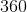## Example Questions

### Example Question #1 : Rectangles

Two rectangles are similar. The perimeter of the first rectangle is 36. The perimeter of the second is 12. If the base length of the second rectangle is 4, what is the height of the first rectangle?

10

6

2

4

8

6

Explanation:

Solve for the height of the second rectangle.

Perimeter = 2B + 2H

12 = 2(4) + 2H

12 = 8 + 2H

4 = 2H

H = 2

If they are similar, then the base and height are proportionally equal.

B1/H1 = B2/H2

4/2 = B2/H2

2 = B2/H2

B2 = 2H2

Use perimeter equation then solve for H:

Perimeter = 2B + 2H

36 = 2 B2 + 2 H2

36 = 2 (2H2) + 2 H2

36 = 4H2 + 2 H2

36 = 6H2

H2 = 6

### Example Question #1 : How To Find If Rectangles Are Similar

Two rectangles are similar. The first rectangle has a perimeter ofand the second has a perimeter of. If the first rectangle has a length of, what the length of the second rectangle?Explanation:

1. Create a proportion comparing the two given rectangles: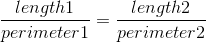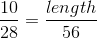2. Solve for the length of the second rectangle by cross-multiplying: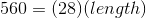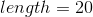### Example Question #1 : How To Find If Rectangles Are Similar

The width of a rectangle is 5 times the length of the rectangle. The width of the rectangle is 30. What is the perimeter of the rectangle?